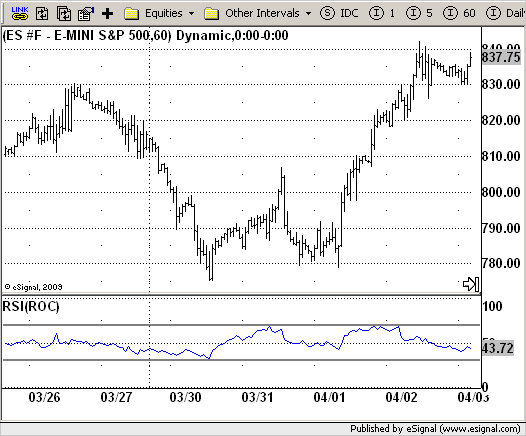# RSI based on ROC

ICE Data Services -

RSI_ROC.efs

EFSLibrary - Discussion Board

File Name: RSI_ROC.efs

Description:
RSI based on ROC

Formula Parameters:

• Length of RSI : 20
• Length of ROC : 20
• Source of ROC : Close
• Upper : 70
• Lower : 30

Notes:
This is the new-age indicator which is version of RSI calculated upon the Rate-of-change indicator.
The name "Relative Strength Index" is slightly misleading as the RSI does not compare the relative strength of two securities, but rather the internal strength of a single security. A more appropriate name
might be "Internal Strength Index." Relative strength charts that compare two market indices, which are often referred to as Comparative Relative Strength.
And in its turn, the Rate-of-Change ("ROC") indicator displays the difference between the current price and the price x-time periods ago. The difference can be displayed in either points or as a percentage. The Momentum indicator displays the same information, but expresses it as a ratio.

RSI_ROC.efsEFS Code:

```/*********************************
Provided By:
eSignal (Copyright c eSignal), a division of Interactive Data
Formula Script (EFS) is for educational purposes only and may be
modified and saved under a new file name.  eSignal is not responsible
for the functionality once modified.  eSignal reserves the right
to modify and overwrite this EFS file with each new release.

Description:
RSI based on ROC

Version:            1.0  04/28/2009

Formula Parameters:                     Default:
Length of RSI                       20
Length of ROC                       20
Source of ROC                       Close
Upper                               70
Lower                               30

Notes:
This is the new-age indicator which is version of RSI calculated upon
the Rate-of-change indicator.
The name "Relative Strength Index" is slightly misleading as the RSI
does not compare the relative strength of two securities, but rather
the internal strength of a single security. A more appropriate name
might be "Internal Strength Index." Relative strength charts that compare
two market indices, which are often referred to as Comparative Relative Strength.
And in its turn, the Rate-of-Change ("ROC") indicator displays the difference
between the current price and the price x-time periods ago. The difference can
be displayed in either points or as a percentage. The Momentum indicator displays
the same information, but expresses it as a ratio.

**********************************/
var fpArray = new Array();
var bInit = false;

function preMain() {
setStudyTitle("RSI(ROC)");
setCursorLabelName("RSI(ROC)",0);
setShowTitleParameters(false);
setDefaultBarFgColor(Color.blue,0);
setStudyMax(101);
setStudyMin(-1);
var x = 0;
fpArray[x] = new FunctionParameter("RSILength", FunctionParameter.NUMBER);
with(fpArray[x++]) {
setName("Length of RSI");
setLowerLimit(1);
setDefault(20);
}
fpArray[x] = new FunctionParameter("ROCLength", FunctionParameter.NUMBER);
with(fpArray[x++]) {
setName("Length of ROC");
setLowerLimit(1);
setDefault(20);
}
fpArray[x] = new FunctionParameter("Upper", FunctionParameter.NUMBER);
with(fpArray[x++]) {
setLowerLimit(0);
setDefault(70);
}
fpArray[x] = new FunctionParameter("Lower", FunctionParameter.NUMBER);
with(fpArray[x++]) {
setLowerLimit(0);
setDefault(30);
}
fpArray[x] = new FunctionParameter("Source", FunctionParameter.STRING);
with(fpArray[x++]){
setName("Source of ROC");
setDefault("close");
}
}

var xRSI = null;

function main(RSILength, ROCLength, Source, Upper, Lower) {
var nBarState = getBarState();
var nRSI = 0;
if (nBarState == BARSTATE_ALLBARS) {
if(RSILength == null) RSILength = 20;
if(ROCLength == null) ROCLength = 20;
if(Source == null) Source = "close";
if(Upper == null) Upper = 70;
if(Lower == null) Lower = 30;
}
if (bInit == false) {